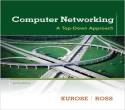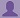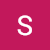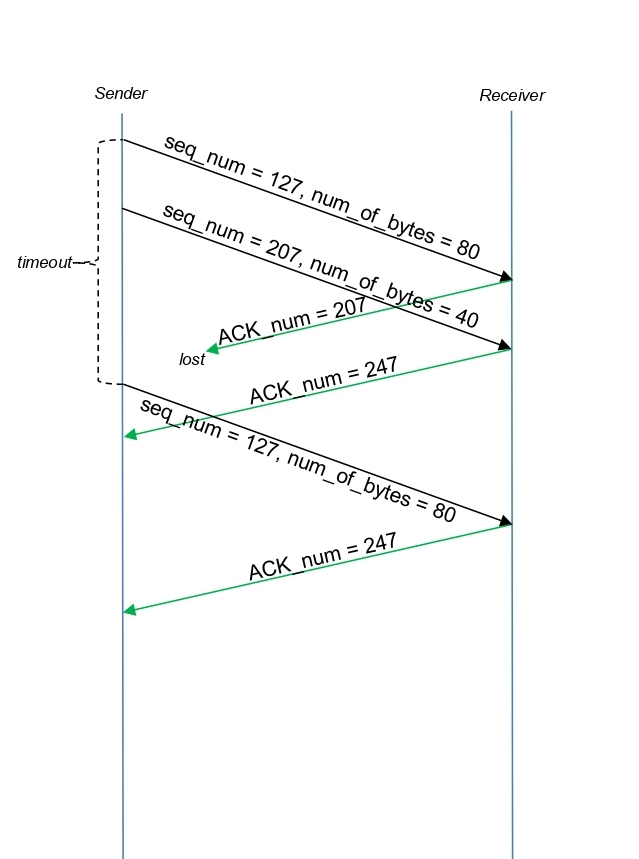SHARE
HELP

To start with, Sr2Jr’s first step is to reduce the expenses related to education. To achieve this goal Sr2Jr organized the textbook’s question and answers. Sr2Jr is community based and need your support to fill the question and answers. The question and answers posted will be available free of cost to all.# Computer Networking : A Top-down Approach

Authors:
James F. Kurose, Keith W. Ross
Exercise:
Problems
Chapter:
Transport Layer
Edition:
6
ISBN:
9780132856201
Question:
27
 Previous Next

###Question

Host A and B are communicating over a TCP connection, and Host B has already received from A all bytes up through byte 126. Suppose Host A then sends two segments to Host B back-to-back. The first and second segments contain 80 and 40 bytes of data, respectively. In the first segment, the sequence number is 127, the source port number is 302, and the destination port number is 80. Host B sends an acknowledgment whenever it receives a segment from Host A.

a. In the second segment sent from Host A to B, what are the sequence num- ber, source port number, and destination port number?

b. If the first segment arrives before the second segment, in the acknowledg- ment of the first arriving segment, what is the acknowledgment number, the source port number, and the destination port number?

c. If the second segment arrives before the first segment, in the acknowl- edgment of the first arriving segment, what is the acknowledgment number? d. Suppose the two segments sent by A arrive in order at B. The first acknowl- edgment is lost and the second acknowledgment arrives after the first time- out interval. Draw a timing diagram, showing these segments and all other segments and acknowledgments sent. (Assume there is no additional packet loss.) For each segment in your figure, provide the sequence number and the number of bytes of data; for each acknowledgment that you add, provide the acknowledgment number.

###Answer

Given data:

• Host A and B are communicating over a TCP connection, and Host B has already received from A all bytes up through byte 126.
• The first and second segments contain 80 and 40 bytes of data, respectively.
• The first segment of sequence number is 127.
• The source port number is 302.
• The destination port number is 80

a)

Sequence number = first segment of sequence number+ destination port number

=127+80

=207

So,  sequence number=207

Source port number = 302

Destination port number= 80

b)

Acknowledgement number= 207

Source port number = 80

Destination port number= 302

c)

Acknowledgement number=127

###Sajidur Rahman

c) If the second segment arrives before the first segment, in the acknowledgement of the first arriving segment, the acknowledgement number will be 127, indicating that it is still waiting for bytes 127 and onwards.

###Stanarth

d) Timeline diagramPost the discussion to improve the above solution.